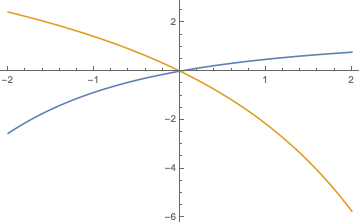# Closed form expression for this infinite series?

Is there a closed-form expression for this series?

$$\displaystyle\sum_{k\geq 1}^\infty \frac{\lambda^k e^{-\lambda}}{k!}\cdot[1-(1-x)^k]\cdot \frac{1}{k}$$

Any answers, ideas or references would be appreciated.

No closed form expression in terms of elementary functions, but if you are satisfied with special functions (incomplete Gamma function $$\Gamma$$ and exponential integral Ei), then $$\displaystyle\sum_{k=1}^\infty \frac{\lambda^k e^{-\lambda}}{k!}\cdot[1-(1-x)^k]\cdot \frac{1}{k}=$$ $$=e^{-\lambda}\,\Re \bigg(\text{Ei}\left(\lambda\right)+\ln (x-1)+\Gamma \left[0,\lambda (x-1)\right]\biggr),\;\;\lambda,x\in\mathbb{R}.$$ ($$\Re$$ indicates the real part.) Here is a plot of the $$x$$-dependence for $$\lambda=+1$$ (blue) and $$\lambda=-1$$ (gold).• well, this would imply that $\Gamma(0,x)$ is an elementary function, which it is not. – Carlo Beenakker Jan 24 '19 at 21:28